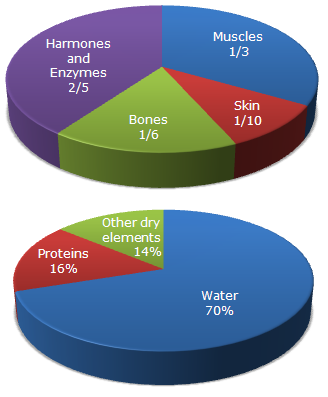# Data Interpretation - Pie Charts - Discussion

Study the following pie-diagrams carefully and answer the questions given below it:

Percentage Composition of Human Body2.

What will be the quantity of water in the body of a person weighing 50 kg?

 [A]. 20 kg [B]. 35 kg [C]. 41 kg [D]. 42.5 kg

Explanation:

 Quantity of water in the body of a person weighing 50 kg = (70% of 50) kg = 35 kg.

 Sara said: (Jul 23, 2017) Can anyone explain this?

 Sammy said: (Nov 7, 2017) Its a biological fact dear, that 70% of human body consist's of water. Hope this helps :).

 Sham said: (Oct 12, 2018) 70/100 x 50 = 7/10 x 50, 7/1 x 5 = 35/1 then 35.